Középiskolai Matematikai és Fizikai Lapok
Informatika rovattal
 Már regisztráltál? Új vendég vagy?# Exercises and problems in Physics March 1999

## New experimental problem:

m. 205. Measure the coefficient of thermal expansion of wood along the grain and perpendicularly to it. (6 points)

## New exercises:

FGy. 3233. A person's heart does approximately a work of 1.6 J during a beat. How much work does it do during a day? How many kg of sand could a machine with such a power output shovel up' to a height of 1 m? (3 points)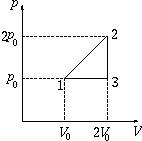FGy. 3234. A certain quantity of gas makes the cycle shown in the figure. The thermodynamic efficiency of the cycle is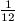. Can this gas be radon? (4 points)

FGy. 3235. Lying on his stomach at the bottom of a slope that has an inclination of 35o, somebody tries to hit the mark 40 metres upward along the slope with a gun. Where should the gun point if the initial speed of the bullet is 100 m/s and air resistance is neglected? (4 points)

FGy. 3236. A body with a mass of 0.5 kg is fastened to the free end of a light, cylindrical rod with a length of 40 cm and a diameter of 0.5 cm. The period of the small oscillations of the system is measured to be 0.1 second. Determine the value of Young's modulus. (5 points)

FGy. 3237. Electrons inside a solenoid revolve along a circular path. In what direction: according or opposite to that of the electrons moving inside the wire of the solenoid? (3 points)

## New problems:

FF. 3238. Two solid, homogeneous spheres of identical weight and radius start down the same slope at the same time, with no initial speed. The quality of the surface of each sphere is different: the coefficient of dynamic friction is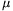1=0.1 for one of them and2=0.4 for the other one.

a) Which sphere arrives first at the bottom of the slope?

b) Which sphere has a greater kinetic energy at the bottom of the slope? Represent these ratios as a function of the inclination of the slope. (5 points)

FF. 3239. We want to tune the E-string of our guitar to the A-string already tuned, applying the usual method: we hold it down so that it also gives an A, then we touch both strings and listen. We can hear the sound fading every second. By how many percents does the frequency of the false A differ from the required value? Is it higher or lower? The frequency of the tuned A-string is 110 Hz. (4 points)

FF. 3240. Estimate the length of time up to which a fire extinguisher with a volume of 10 litres, containing 4 kg of carbon dioxide can be operated. Assume that the gas leaves the container through a hole with a cross-section of 0.1 cm2, at a uniformly decreasing speed. (5 points)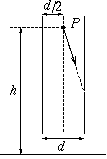FF. 3241. A particle of charge Q and mass m is released from point P inside a vertical plane capacitor charged to pd. U, as shown in the figure. There is a small hole at the point where the particle reaches the right plate, through which the particle can pass. What is the trajectory of the particle? In how much time and where does the particle reach the ground? (Air resistance can be neglected.) (4 points)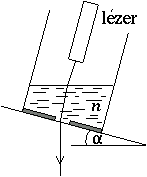FF. 3242. A recently patented plummet' consists of a liquid with a suitable refractive index poured into a cylindrical vessel and of a small laser. How should the refractive index n be chosen so that the light refracted by the liquid prism leaves the apparatus practically vertically when |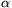| <10o? How accurate can this apparatus be? (5 points)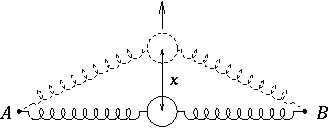FN. 3243. A body of mass m can move without friction in a horizontal plane. The springs fixed in points A and B shown in the figure are initially not expanded, their spring constant is D.